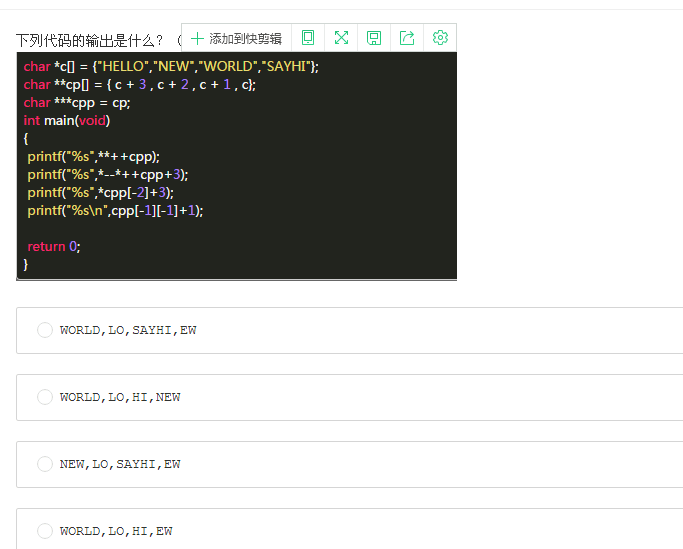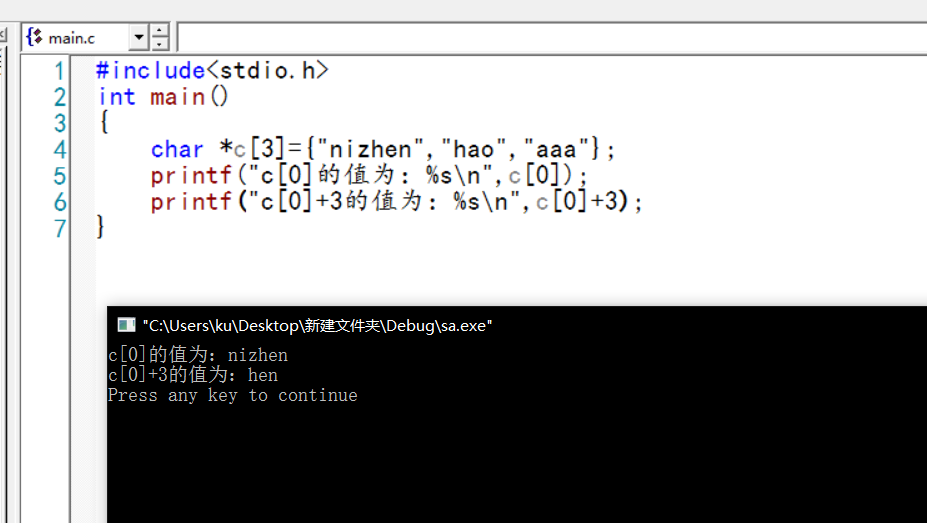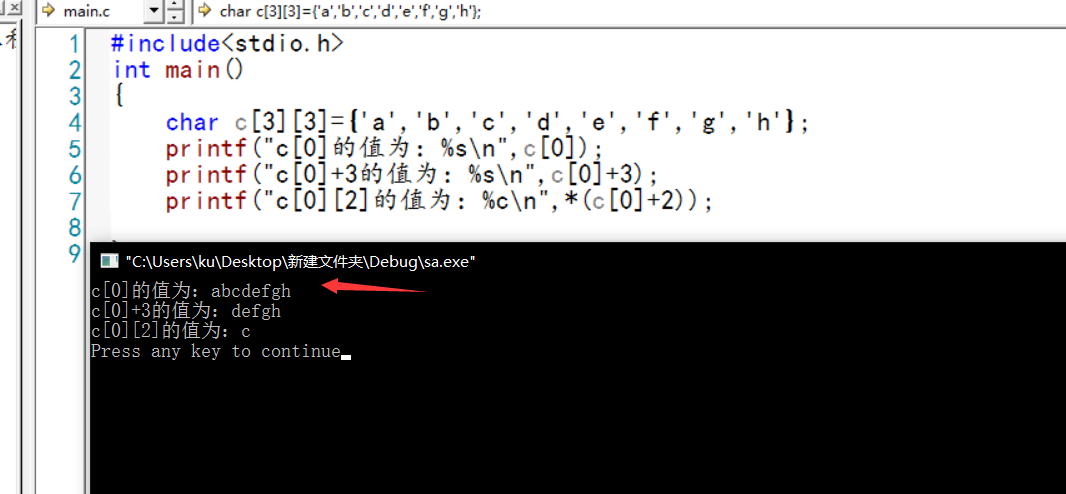• ## C语言指针例题

千次阅读 多人点赞 2019-06-22 20:51:38
指针方法编写一个程序，输入3个整数，将它们按由小到大的顺序输出 #include <stdio.h> void swap(int *pa,int *pb) { int temp; temp = *pa; *pa = *pb; *pb = temp; } void main() { ...
用指针方法编写一个程序，输入3个整数，将它们按由小到大的顺序输出
    #include <stdio.h>
void swap(int *pa,int *pb)
{
int temp;
temp = *pa;
*pa = *pb;
*pb = temp;
}
void main()
{
int a,b,c,temp;
scanf("%d%d%d",&a,&b,&c);
if(a>b)
swap(&a,&b);
if(b>c)
swap(&b,&c);
if(a>c)
swap(&a,&c);
printf("%d,%d,%d",a,b,c);
}

用指针方法 输入3个字符串 按由小到大顺序输出
    #include "stdio.h"
#include "string.h"
int main(int argc, char* argv[])
{
char *t;
char *p1=NULL,*p2=NULL,*p3=NULL;
char ch1={0},ch2={0},ch3={0};

p1=ch1;
p2=ch2;
p3=ch3;

printf("No1:");
scanf("%s",p1);
fflush(stdin);
printf("No2:");
scanf("%s",p2);
fflush(stdin);
printf("No3:");
scanf("%s",p3);
fflush(stdin);

if(strcmp(p1,p2)>0)
{t=p1;p1=p2;p2=t;}

if(strcmp(p1,p3)>0)
{t=p1;p1=p3;p3=t;}

if(strcmp(p2,p3)>0)
{t=p2;p2=p3;p3=t;}

printf("%s\n%s\n%s\n",p1,p2,p3);

return 0;
}

编程输入一行文字，找出其中的大写字母，小写字母，空格，数字，及其他字符的个数
    #include<stdio.h>
void main()
{
int a=0,b=0,c=0,d=0,e=0,i=0;
char *p,s;
while((s[i]=getchar())!='\n')i++;
p=s;
while(*p!=10)
{
if(*p>='A'&&*p<='Z')
a++;
else if(*p>='a'&&*p<='z')
b++;
else if(*p==' ')
c++;
else if(*p>='0'&&*p<='9')
d++;
else e++;
p++;
}
printf("大写字母 %d 小写字母 %d\n",a,b);
printf("空格 %d 数字 %d 非字符 %d\n",c,d,e);
}

写一个函数，将3 3矩阵转置
    #include "stdio.h"

void Transpose(int (*matrix))
{
int temp;
int i, j;

for(i=1;i<3;i++)/*转置*/
{
for(j=0;j<i;j++)
{
temp = *(*(matrix+j)+i);
*(*(matrix+j)+i) = *(*(matrix+i)+j);
*(*(matrix+i)+j) = temp;
}
}
}

void main()
{
int a = {{1,2,3},{4,5,6},{7,8,9}};
Transpose(a);
for(int i = 0;i<3;i++)
{
for(int j=0;j<3;j++)
{
printf("%d ",a[i][j]);
}
printf("\n");
}
}
}

用指向一维数组的指针做函数参数
    #include<stdio.h>
#include<string.h>
int main()
{
void sort(char (*s));//一维数组的指针做函数参数
int i;
char str;
char (*p);//定义一维数组的指针做函数参数
printf("please input string:/n");
for(i=0;i<10;i++)
scanf("%s",&str[i]);
p=str;//将str一维数组指针，赋值给p;
sort(p);
printf("the output sequence :/n");
for(i=0;i<10;i++)
printf("%s/n",str[i]);
return 0;
}
void sort(char (*s))//s指向一维数组的指针做函数参数；
{
int i,j;
char temp, *t;
t=temp;
for(i=0;i<9;i++)//i应该小于9；如果小于10，那么就比较了9+1次；按照冒泡法则，
for(j=0;j<9-i;j++)//第一次比较需要9次就是i=0到i=8共九次；第二次需要比较8次；依次类推；
if(strcmp(s[j],s[j+1])>0)
{
strcpy(t,s[j]);
strcpy(s[j],s[j+1]);
strcpy(s[j+1],t);
}
}

用指针数组在主函数中输入十个等长的字符串。用另一函数对它们排序，然后在主函数中输出10个已排好序的字符串
    //用指针数组处理
#include<stdio.h>
#include<string.h>
int main()
{
void sort(char *[]);
int i;
char str, *p;
printf("please input 10 string:/n");
for(i=0;i<10;i++)//首先将10个str的首地址赋值给10个p[i];
p[i]=str[i];//将第i个字符串的首地址赋予指针数组p的第i个元素；
for(i=0;i<10;i++)
scanf("%s",p[i]);//scanf输入到&p[i]
sort(p);
printf("the output 10 string:/n");
for(i=0;i<10;i++)
printf("%s/n",p[i]);//输出到p[i];
}
void sort(char *s[])
{
char *temp;
int i,j;
for(i=0;i<9;i++)
for(j=0;j<9-i;j++)
if(strcmp(*(s+j),*(s+j+1))>0)
{
temp=*(s+j);//*(s+j)指向数组指针,我想应该是字符串的首地址；所以可以直接赋值给temp指针；
*(s+j)=*(s+j+1);
*(s+j+1)=temp;
}
}

指针 将n个数按输入时顺序的逆序排列,用函数实现
    #include <stdio.h>
void reverse(int a[],int n)
{ int *p;
for(p=a+n-1;p>=a;p--)
printf("%4d",*p);
printf("\n");
}
main()
{ int a,n;
int i;
printf("Input the length of array:");
scanf("%d",&n);
printf("Input the number of array:");
for(i=0;i<n;i++)
scanf("%d",&a[i]);
reverse(a,n);
}

写一函数，实现两个字符串的比较。即自己写一个strcmp函数，函数原型为：
int stremp(char *p1,char *p2)
设p1指向字符串s1，p2指向字符串s2。要求：当s1=s2时，返回值为0。当s1不等于s2时，返回它们二者的第一个不同字符的ASCII码差值（如“BOY”与“BAD”，第二字母不同，“O”与“A”之差为79-65=14）；如果s1>s2，则输出正值；如果s1<s2，则输出负值。
    #include <stdio.h>
main()
{ int strcmp(char *p1,char *p2);
int m;
char str1,str2,*p1,*p2;
printf("Input two strings:\n");
scanf("%s",str1);
scanf("%s",str2);
p1=&str1;
p2=&str2;
m=strcmp(p1,p2);
printf("result: %d\n",m);
}

int strcmp(char *p1,char *p2)            /*两个字符串比较的函数*/
{ int i;
i=0;
while(*(p1+i)==*(p2+i))
if(*(p1+i++)=='\0') return(0);         /*相等时返回结果0*/
return(*(p1+i)-*(p2+i));     /*不等时返回结果为第一个不等字符ASCII码的差值*/
}

运行情况如下：
① Input two strings:
CHINA↙
Chen↙
Result: -32
② Input two strings:
hello! ↙
Hello! ↙
Result: 0
③ Input two stings:
dog↙
cat↙
result: 1
编一个程序，打入月份号，输出该月的英文月名。例如，输入“3”，则输出“March”，要求用指针数组处理。
    #include <stdio.h>
main()
{ char *month_name={"illegal month","January","February","March","April",
"May","June","July","August","September","October","November","December"};
int n;
printf("Input month: ");
scanf("%d",&n);
if((n<=12)&&(n>=1))
printf("It is %s.\n",*(month_name+n));
else
printf("It is wrong.\n");
}

运行结果：
① Input month: 2↙
It is February.
② Input month: 8↙
It is August.
③ Input month: 13↙
It is wrong.
将字符串computer赋给一个字符数组，然后从第一个字母开始间隔地输出该串。请用指针实现
    #include <stdio.h>
#include <string.h>
#define MAX_LENGTH 32

int main()
{

char str[MAX_LENGTH] = {0};
char *pStr = (char*)&str;

//1.  将字符串computer赋给一个字符数组
strcpy(str, "computer");

//2. 然后从第一个字母开始间隔地输出该串
while(*pStr != '\0' )
{
printf("%c\n", *pStr);
pStr++;
}

return 1;
}

从键盘上输入一个字符串，按后按照下面要求输出一个新字符串， 新的字符串是在原来字符串中， 每两个字符之间插入一个空格， 如原来的字符串为“abcd”， 新产生的字符串应为“a b c d”
编写一个程序咯 用C 就是输出的字符 是 每两个字符之间有一个空格
#include<stdio.h>
void main()
{
char a,b,i=0,j=0;
printf("输出字符串");
scanf("%s",a);// abcdef
for(i=0;a[i]!='\0';i++)
{
b[j++]=a[i];
b[j++]=' ';
}
b[j]='\0';
printf("%s",b);
}

设有一数列，包含10 个数，已按升序排好。现要求编一程序，它能够把从指定位置开始的n个数按逆序重新排列并输出新的完整数列。进行逆序处理时要求使用指针方法（例如：原数列为2，4，6，8，10，12，14，16，18，20，若要求把从第4个数开始的5个数按逆序重新排列，则得到新数列为2，4，6，16，14，12，10，8，18，20。）
    #include <iostream.h>

void  method(int n,int m,int *a)
{
int *p=a,*q=new int[m];

p=p+n-1;
for(int i=0;i<m;i++)
{
q[i]=*p;
p++;
}
p=p-m;
for(i=0;i<m;i++)
{
*p=q[m-1-i];
p++;
}
}

int main()
{
int a={2,4,6,8,10,12,14,16,18,20};
method(4,5,a);
for(int i=0;i<10;i++)
{
cout<<a[i]<<' ';
}
return 0;
}

有一字符串，包含n个字符。写一个函数，将此字符串从第m个字符开始的全部字符复制成为另一个字符串并输出
    void strcpyn(char *s,char *t, int n)
{
char *p=s+n;
char *q=t;
while(*p)
{
*q=*p;
q++;
p++;
}
*q='\0';

}
int main()
{
char s={0};
char t={0};
int n=0;
printf("input string s:\n");
scanf("%s",s);
printf("input start n:\n");
scanf("%d",&n);
strcpyn(s,t,n);
puts(t);
}

设计函数char *insert(s1,s2,n),用指针实现在字符串s1中的指定位置n处插入字符串s2
#include <stdio.h>

char* insert(char* s1,char* s2,int n)
{
int j=0;
char* ss=new char;
char* tsptr=ss;

for(int i=0;i<n;i++)
*ss++=*s1++;
while(*s2!='\0')
*ss++=*s2++;
while(*s1!='\0')
{
*ss++=*s1++;
}
*ss='\0';
return tsptr;
}
void main()
{
char s1[]="123456789";
char s2[]="1234";
char* ss=insert(s1,s2,4);
printf("%s",ss);
}

利用指针完成字符串复制函数char *strcpy(char *s1,char *s2)
#include "stdio.h"
char* fun(char *s1,char *s2)
{
char *p=s2;
while((*s1)!='\0')
{
*s2=*s1;
s1++,s2++;
}
return p;
}
void main(void)
{
char *p,*strcpy;
char *s1="world cup!";
char *s2="good luck!";
printf("s1=%s\ns2=%s\n",s1,s2);
p=fun(s1,s2);
printf("s1=%s\ns2=%s\n",s1,s2)
}


编写一个使用指针的c函数，交换数组a和数组b中的对应元素

    #include<stdio.h>
#include<stdlib.h>

void ex(int *a, int *b, int n){
int i,tmp;
for (i=0;i<n;i++){
tmp=a[i];a[i]=b[i];b[i]=tmp;
}
}

void main()
{
int x={1,2,3,4,5};
int y={6,7,8,9,10};
int i,n=5;
ex(x,y,n);
printf("\n X: ");
for (i=0;i<n;i++)printf("%d ",x[i]);
printf("\n Y: ");
for (i=0;i<n;i++)printf("%d ",y[i]);
system("pause");
}



展开全文• ## c语言指针例题

千次阅读 2013-03-18 21:07:21
（3）在第（2）小题的基础上，保持reverse函数不改动，将主程序中实参改为采用指针变量形式实现 void reverse(int *p)  {  int t,i,j;  for (i=0,j=N-1;i;i++,j--)  {  t=*(p+i);  *(p+i)=*(p+j...
第一题：
int main() {double salarys = { 1500, 2000.43, 1202.78, 8000.32, 5000 };int i = 0;for (i = 0; i < 5; i++) {printf("%6.2lf\n", salarys[i]);}int *p;p = &salarys;int *m;m = &salarys;printf("%x\n", p);printf("%x\n", m);int *n;n = &salarys;printf("%x\n", n);printf("%x\n", &salarys);double *head;head = &salarys;double *tail = &salarys;printf("%x\n", head);printf("%x\n", tail);printf("%6.2lf\n", *head);printf("%6.2lf\n", *tail);printf("%x\n", tail - head);*head = salarys - 50;printf("%6.2lf\n", *head);printf("%6.2lf\n", *head + 6550.32);head = &salarys;for (i = 0; i < 5; i++) {printf("%6.2lf\n", *(head + i));}tail = &salarys;for (i = 4; i >= 0; i--) {printf("%6.2lf\n", *(tail + i));}return EXIT_SUCCESS;
}
第二题：
编写一个函数，此函数能够接收下面所有数据类型变量的地址，并能够在函数中将其值打印。
int main(){
int a = 1;
char c = 'c';
float f = 11.11;
double d = 22.222;
void *p,*p1,*p2,*p3;
p = &a;
printf("%d\n", *((int*)p));
p1 = &c;
printf("%d\n", *((char*)p1));
p2 = &f;
printf("%2.2lf\n", *((float*)p2));
p3 = &d;
printf("%2.4lf\n", *((double*)p3));
return EXIT_SUCCESS;
}
第三题：
从键盘输入一个字符串，按照字符顺序从小到大进行排序，并要求删除重复
的字符。
void f(char *str) {
char *p, *q, *r, c;
for (p = str; *p; p++) {
for (r = p, q = p + 1; *q; q++)
if (*r > *q)
r = q;
if (r != p) {
c = *r;
*r = *p;
*p = c;
}
}
for (p = str; *p; p++) {
for (q = p + 1; *q && *q == *p; q++)
strcpy(p, q--);
}
}
int main() {
char s;
printf("请输入一个字符串:\n");
fflush(stdout);
gets(s);
f(s);
printf("结果为：\n%s\n", s);
return EXIT_SUCCESS;
}
第四题：
有n 个人围成一个圆圈，顺序排号，从第一个人开始报数(从1 到3 报数)，
凡报到3 的人退出圈子，问最后留下的是原来的第几号？
int i, k, m, n, num, *p;
printf("请输入一共有多少人：\n");
fflush(stdout);
scanf("%d", &n);
p = num;
for (i = 0; i < n; i++)
*(p + i) = i + 1;
i = 0;
k = 0;
m = 0;
while (m < n - 1) {
if ((*(p + i)) != 0) {
k++;
}
if (k == 3) {
*(p + i) = 0;
k = 0;
m++;
}
i++;
if (i == n)
i = 0;
}
while (*p == 0)
p++;
printf("最后留下的是原来的第%d号！\n", *p);
第五题：
编写一个函数findstr(char
*str,char *substr)，该函数统计一个长度为2 的子字符串在另一个字符串中出
现的次数。
int findStr(char *str, char *substr) {
int cnt = 0;
while (*str != '\0') {
if (*substr != *str) {
str++;
continue;
} else if (*substr == *str) {
str++;
substr++;
if (*substr == *str) {
cnt++;
str++;
substr--;
} else {
substr--;
continue;
}
}
}
return cnt;
}
int main() {
char str1, str2, c;
printf("输入原字符串:\n");
fflush(stdout);
gets(str1);
printf("输入子字符串:\n");
fflush(stdout);
gets(str2);
c = findStr(str1, str2);
printf("子字符串在原字符串中出现的次数为：\n%d次！\n", c);
return 0;
}
第六题：
编写程序实现如下功能：输入一个整数字符串转换为一个整数值，如”1234”
转换为1234，”-1234”转换为-1234。
int main(void) {
char s;
int n;
long chnum(char *p);
printf("输入一个字符串:\n");
fflush(stdout);
gets(s);
if (*s == '-')
n = -chnum(s + 1);
else
n = chnum(s);
printf("将一个整数字符串转换为一个整数值为：\n%d\n", n);
return 0;
}
long chnum(char *p) {
long sum = 0;
while (*p) {
if (*p >= '0' && *p <= '9')
sum = sum * 10 + *p - '0';
p++;
}
return sum;
}
第七题：
void IntPoint(int * s, int * e, int i) {
while (s != e) {
*s = i;
s++;
}
*e = i;
}
int main() {
int a = { 0 };
int i;
IntPoint(a, a + 9, 10); // 尾指针为 a + 9 ，不是 a + 10
for (i = 0; i < 10; i++) {
printf("%d ", a[i]);
}
return 0;
}
第八题：
从键盘输入一个n×n 的二维数组（n 键盘输入），找出此二维数组中各行的
最大值，并按从大到小的次序输出各行的最大值及此值所在的行号
int main(void)
{
int a,b,c;//定义b数组保存各行最大值，c数组保存各行行号
int n,i,j,k=0,l=0,index,max,temp;
scanf("%d",&n);
printf("请输入%d阶矩阵:",n);
for(i=0;i<n;i++)
for(j=0;j<n;j++)
scanf("%d",&a[i][j]);
for(i=0;i<n;i++)
{
max=a[i];
for(j=1;j<n;j++)
if(a[i][j]>max) max=a[i][j];
b[k++]=max;//记录此行的最大值
c[l++]=i+1;//记录这时的行号
}
for(i=0;i<n-1;i++)//选择排序
{
index=i;
for(j=i+1;j<n;j++)
if(b[j]>b[index]) index=j;
if(index==i) continue;
temp=b[index];
b[index]=b[i];
b[i]=temp;
temp=c[index];//交换最大值时别忘了交换相应的行号
c[index]=c[i];
c[i]=temp;
}
printf("每行最大数排序后：\n");
for(i=0;i<n;i++)
printf("%-4d在第%2d行\n",b[i],c[i]);
return 0;
}
第九题：
（2）将reverse函数形参改为采用指针变量形式实现
void reverse(int *p) {
int t, i, j;
for (i = 0, j = N - 1; i < j; i++, j--) {
t = *(p + i);
*(p + i) = *(p + j);
*(p + j) = t;
}
}
int main() {
int i, b[N] = { 98, 76, 68, 65, 57, 53, 45, 43, 32, 29 };
reverse(b);
for (i = 0; i < N; i++)
printf("b[%d]=%d\n", i, b[i]);
return EXIT_SUCCESS;
}
（3）在第（2）小题的基础上，保持reverse函数不改动，将主程序中实参改为采用指针变量形式实现
void reverse(int *p)
{
int t,i,j;
for (i=0,j=N-1;i<j;i++,j--)
{
t=*(p+i);
*(p+i)=*(p+j);
*(p+j)=t;
}
}
int main()
{
int i, b[N]={98,76,68,65,57,53,45,43,32,29};
int *a=b;
reverse(a);
for (i=0;i<N; i++)
printf("b[%d]=%d\n", i, b[i]);
return EXIT_SUCCESS;
}

展开全文• 10.5 指针与字符串 字符串表示形式 用字符数组实现 例 main( ) { char string[]=I love China; printf%s\n,string; printf%s\n,string+7; } I l o v e C h i string string string string string ...
• ## c语言指针例题解答

万次阅读 多人点赞 2017-10-18 00:21:30
首先char*c[]为一个指针数组，对吧，这个相信大家都能看懂，千万不要把指针数组和数组指针搞混乱了。 首先先给大家讲解下什么是指针数组，什么又是数组指针呢？ eg:char *c 因为[]的优先级高于*，所以c先与[]...


这个文字排版我都看不下去了，点击新连接，前去新排版------->>点击此处

ps: 新排版更适合阅读呢~

---首先char*c[]为一个指针数组，对吧，这个相信大家都能看懂，千万不要把指针数组和数组指针搞混乱了。

首先先给大家讲解下什么是指针数组，什么又是数组指针呢？

eg:char *c 因为[]的优先级高于*，所以c先与[]结合形成一个数组，类型为char* 类型，大小为5。里面存放属于char类型的指针。其实数组指针和二维指针有一定的相似之处，如果感兴趣，大家可以百度什么是二维指针，在以后的篇幅里，我也会详细给大家解释什么是二维指针，他们的联系是什么，区别又是什么呢？

而对于char(*c) 因为（）的优先级最高，所以c先和*形成一个指针，然后再与数组结合，这就形成了数组指针，即为指向数组的指针。它指向包含5个char类型元素的一维数组，此时c的增量以它所指向的一维数组长度为单位；

好了 弄懂了数组指针和指针数组，那么接下来继续解答该道题。

看这句代码，char**cp[]={c+3,c+2,c+1,c};

仔细看看，这里的**cp[]比上面的*c多了一个*。不知道大家看到这里有没有点疑惑呢？

多一个*号那他又代表什么呢？我来给大家举一个简单的例子吧。

char a='a';

char *b=&a;

上面这个代码的很简单吧！先定义了一个char变量,再由b指向a的地址。也就是说b里面存放的数据是变量a的地址。那么接下来就简单了，

再回到原题，char**cp[]也比char*c[]多一个*,那么不用说，cp[]里面也是存放着c[]的地址吧。看看cp[]里面的元素，c+3,c+2...果然是地址吧。

这里大家又有疑问了，为什么c+3是一个地址？那你们听说过 “数组名可看做一个地址”这句话吧！，那就对了，虽然c[]是个指针数组，那他仍然是个数组啊!!这就不难理解c+3,c+2等地址了吧。这里大家谨记可以吧数组名看做指针，但是数组名与指针又不是完全等价的！这个以后慢慢详解。那么接下来回到原题，看第三句，char***cpp=cp;

这句话我想很好理解吧！他是把一个存放指针数组地址的数组显式转化为一个指针。emmmmm，是不是有点头晕，转化来转化去的。看看他有3个*号，你就知道他是个三级指针了。

接下来看第一句printf， 参数是**++cpp;首先大家要知道输出三级指针指向的内容，他两个*又代表什么。

首先先看cpp前面有个++,什么意思呢？大家看看第三句代码，cpp指向cp，那么++cpp是不是就是cp的地址啊，肯定是的。然后带个*号，取到cp的值，而cp的值是c+2，同时c+2又是一个地址，请大家好像体会这句话，给一个指针变量前面加一个*号就是表示他指针指向地址的值，那么这就很好理解**++cpp了。先是++cpp获取到cp的地址，要获取cp指向的内容再给前面加个*号就可以了，即*++cpp 就代表cp,即c+2。而c+2也是一个地址，要获取到c+2这个地址指向的内容，那再给前面加个*号就可以了。即为**++cpp;

这样**++cpp就代表指针数组c的第三个元素了，是一个字符串“world”,%s接收到这个字符串会把它都输出出来。即第一个printf打印WORLD.

举个简单的例子，给你们提个问题。

int a={1,2,3,4,5}

int *p=a;

p++;//1

a++；//2

这两个哪个正确呢？

1是正确的2是错误的。

要取到里面的元素  *(p),*(p+1)......;注意看这里也带个*，这个代码的意义是把数组显式转化为一个指针！，再结合图片的第二行和第三行，你现在应该清楚他的作用了吧。对，就是将数组显式转化为一个同维度的指针。。。好累明天继续更博。

接下来回到问题，因为经过第一句**++cpp完后，cpp已经指向了cp，再次的*++cpp同样的道理，获取到cp的元素，即c+1这个地址。然后再--,即为c这个地址，再带个*号，即为c这个地址下代表的值，其实也可以这么说，可以把指针数组看成一个二维数组。可以写成这样c。那么c此时代表的值应该是二维数组的第一行吧。即c。即此时为“hello”,别忘记了，后面还有个+3呢。因为hello本身也是个地址，如果大家再此又困惑，我给大家举了一个简单的例子。希望大家能认真看下帮助理解。%s这个格式控制符需要接收一个字符串地址，c地址获取到了，他就会把c输出，这里给大家提个问题。他获取到c地址后，会不会也把c也输出出来呢？

哈哈  ，不要困惑了。并不会的。因为c这个字符串“hello\0”，他会有个默认的'\0'结束符，结束%s的获取。我为什么会问这样的问题。因为要是同样的问题 遇到了二维数组大家想想又是什么样的情况呢？大家好好对比看下。针对二维数组,让%s获取到c即二维数组的第一行 时，他会把整个二维数组输出出来。直到遇到c是个默认的‘\0’结束输出。

通过一个例题 我举出这么多例子。。。。真累。不知道大家能不能理解呢？

接下来回到问题的第三个输出，参数为*cpp[-2]+3。不难理解。可以转化下，切记接下来这个让我受益终身的一句语法糖，*（a+1）等价a。当你被指针搞得头大恨不得疯掉的时候，一定要想起这句语法糖。我不扯了。回到原题 。根据语法糖法则，把*cpp[-2]+3转化成**(cpp-2)+3。我想通过我这么详细的分析。这道题对大家不再有难度了吧。算了 ，送佛送到西。cpp经过上面两句已经指向了cp这个地址，那么cpp-2指向cp的地址没疑惑吧。然后带个*，即为*（cpp-2）即为cp这个元素，即代表c+3这个地址,然后再带个*号为**（cpp+3）,成为c代表的元素。c+3这个地址代表的字符串是“SAYHI”，再+3,即把该字符串的地址再往后移动三位。这样就输出了“HI”。切记*（地址）=该地址指向的值。这句话可以套用。即就是这里面的地址可以是地址的地址。请各位好好理解吧。eg：*（*（地址的地址））

接下来第四句 cpp[-1][-1]大声告诉我，是什么！！！算了 ，我继续解答吧，谁让我就是这么这么好呢。老规矩，先转化成*（*（cpp-1）-1）+1；你们说下cpp此时指向谁呢？指向cp，如果你觉得我说错了，请好好回头仔细看看。很多人困惑了，为什么第三句输出不是移动了cpp了啊 。请你再仔细看看第一句，第二句和第三句printf里面的区别。对的，第一句第二句++cpp和第三句cpp-2是完全不相同的道理。就比如int a=1；a++和a+2能一样吗？显然是不一样的 a++后a自增为2。a+2执行完后a还是1。好了接下来回到原题。指向cp后带个*号取到cp的元素即为c+2又是个地址，在把这个地址--,得到c+1的地址。再带*号，得到c+1地址指向的值。为“New”，得到这个字符串地址后，再+1,输出EW。

此题结束 。答案选哪个呢？

我再问大家一个问题还是原来的那些数组，删掉那4句printf，重新写一句printf(“%c”,cpp)输出什么呢？ 我给个答案 ：输出A。

printf(“%c”,cpp[-1])又输出多少呢？答案：输出E。

请大家好好想想吧!指针的道路就是这样一步一步探索吧。我相信各位勇于尝试最终都能成为C指针高手。

我才疏学浅，如若在我的博客里发现错误，请私信或评论，我会积极修改的。我知道CSDN博客里藏龙卧虎，还望大家不吝赐教。第一次写博客，乱七八糟的，希望大家多多宽恕。

展开全文• 题目： 有n个整数，使前面各数顺序向后移m个位置，最后m个数变成最前面m个数。 #include int main() {  void move(int ,int,int);  int number,n,m,i;  printf("how many numbers?...
题目： 有n个整数，使前面各数顺序向后移m个位置，最后m个数变成最前面m个数。

#include <stdio.h>
int main()
{
void move(int ,int,int);
int number,n,m,i;
printf("how many numbers?");
scanf("%d",&n);
printf("input %d numbers:\n",n);

for(i=0;i<n;i++)
scanf("%d",&number[i]);
printf("how many place you want move?");
scanf("%d",&m);
move(number,n,m);
printf("Now,they are:\n");
for(i=0;i<n;i++)
printf("%d ",number[i]);
printf("\n");
return 0;
}

void move(int array,int n,int m)
{
int *p,array_end;
array_end=*(array+n-1);  // 把原数组中最后一个数赋值给array_end。 这里的*(array+n-1)就等价于array[n-1];
for(p=array+n-1;p>array;p--){
*p=*(p-1);                 //这个循环函数的功能是把数组中所有的数向后移动一位。printf("%d ",*p);}printf("\n");printf("%d \n",array_end);
*array=array_end;          //把之前数组中最后一个数赋值给移动后第一位空缺的位置。 array是数组名，*array就代表数组的第一位。printf("%d \n",*array);
m--;                       // 因为移动m位，所以要迭代m次。
if(m>0)
move(array,n,m);
}  `
展开全文• ## C语言经典指针例题

千次阅读 2018-11-23 11:55:31
经典指针例题： main(){ int va,vb,*pa,*pb,i; pa=va; pb=vb; for(i=0;i&lt;3;i++,pa++,pb++){ *pa=i; pb=2i; printf("%d\t%d\n",*pa,*pb); } pa=&amp;va; pb=&amp;vb; printf...
• 指针例题 指针加减整数 由例题可以知道，指针的类型决定指针能走多长。 指针不仅有数组指针，函数指针，还有更为复杂的指向函数指针的数组的指针。能够学好指针就能把C语言用好用活。 笔试题解析 指针...函数
• //要求：利用字符串指针指针数组完成。 char *array={"yangmufa","shuai","de","chaojishuai",}\ ,(*tem)=NULL,t={NULL}; int i=0,j=0,k=0; for(i=0;i;i++){//i表示从第一个开始到倒数第二个结束(4个...
• ## C语言的指针例题

千次阅读 2013-03-15 09:06:03
（1）定义一个double类型的指针head，并初始化为NULL。 （2）定义一个double类型的变量salary，并初始化为8000.75，让head指向salary。 （3）使用&显示变量salary所占内存的地址值。 （4）使用head显示salary所占...
• 例1-写一个函数，输入一行字符，将此字符串中最长的单词输出。 例2-建立一个对象数组，内放5个学生的数据（学号、成绩），用指针指向数组首元素，输出第一、三、五个学生的数据。
•c...

# c语言指针例题c语言 订阅